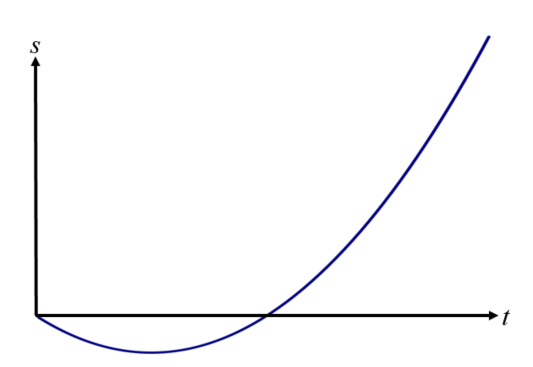# Exam-Style Question on Kinematics

## A mathematics exam-style question with a worked solution that can be revealed gradually

##### List Of QuestionsExam-Style QuestionMore Kinematics QuestionsOther Topics

Question id: 573. This question is similar to one that appeared on an IB AA Standard paper in 2021. The use of a calculator is not allowed.

A particle travels in a straight line such that its displacement, $$s$$ metres, from a fixed point, $$A$$, after $$t$$ seconds is given by $$s(t) = t^2 - 5t$$, for $$0 \le t \le 10$$, as shown in the following sketch.The particle starts at $$A$$ and passes through $$A$$ again when $$t = q$$.

(a) Find the value of $$q$$.

The particle changes direction when $$t = r$$.

(b) Find the value of $$r$$.

(c) Find the displacement of the particle from $$A$$ when $$t = r$$.

(d) Find distance of the particle from $$A$$ when $$t=10$$.

(e) Find the total distance travelled by the particle in ten seconds.

A second particle travels along the same straight line such that its velocity is given by $$v(t)=12 - 3t$$ for $$t \ge 0$$.

When $$t = p$$, the distance travelled by this second particle is one metre less than the total distance travelled by the first particle in ten seconds.

(f) Find the value of $$p$$

 The worked solutions to these exam-style questions are only available to those who have a Transum Subscription. Subscribers can drag down the panel to reveal the solution line by line. This is a very helpful strategy for the student who does not know how to do the question but given a clue, a peep at the beginnings of a method, they may be able to make progress themselves. This could be a great resource for a teacher using a projector or for a parent helping their child work through the solution to this question. The worked solutions also contain screen shots (where needed) of the step by step calculator procedures. A subscription also opens up the answers to all of the other online exercises, puzzles and lesson starters on Transum Mathematics and provides an ad-free browsing experience.Drag this panel down to reveal the solution

The exam-style questions appearing on this site are based on those set in previous examinations (or sample assessment papers for future examinations) by the major examination boards. The wording, diagrams and figures used in these questions have been changed from the originals so that students can have fresh, relevant problem solving practice even if they have previously worked through the related exam paper.

The solutions to the questions on this website are only available to those who have a Transum Subscription.

Exam-Style Questions Main Page

Search for exam-style questions containing a particular word or phrase:

To search the entire Transum website use the search box in the grey area below.